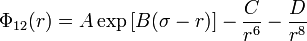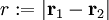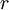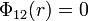2022: SklogWiki celebrates 15 years on-line

# Born-Huggins-Meyer potential

The Born-Huggins-Meyer potential (although looking at the authors/publications perhaps it would be more precisely known as the Born-Meyer-Huggins potential)    is given by$\Phi_{12}(r) = A \exp \left[ B(\sigma - r) \right] - \frac{C}{r^6} - \frac{D}{r^8}$

where

•$r := |\mathbf{r}_1 - \mathbf{r}_2|$
•$\Phi_{12}(r)$ is the intermolecular pair potential between two particles or sites
•$\sigma$ is the diameter (length), i.e. the value of$r$ at which$\Phi_{12}(r)=0$

The first term is an exponential repulsion, followed by dipole-dipole and dipole-quadrupole dispersion terms. This potential is often augmented with a Coulombic interaction.

This potential is often used to study alkali halides.